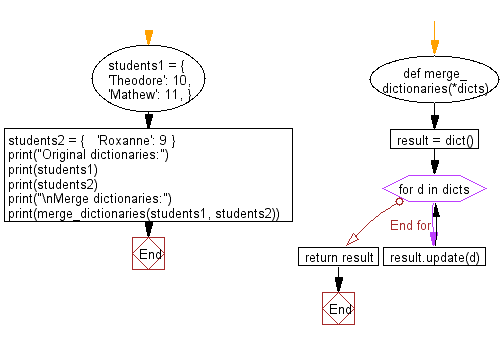﻿ Python: Merge two Python dictionaries - w3resource# Python: Merge two Python dictionaries

## Python dictionary: Exercise-8 with Solution

Write a Python script to merge two Python dictionaries.

Sample Solution-1:

Python Code:

``````d1 = {'a': 100, 'b': 200}
d2 = {'x': 300, 'y': 200}
d = d1.copy()
d.update(d2)
print(d)
```
```

Sample Output:

```{'x': 300, 'y': 200, 'a': 100, 'b': 200}
```

## Visualize Python code execution:

The following tool visualize what the computer is doing step-by-step as it executes the said program:

Sample Solution-2:

Create a new dict and loop over dicts, using dictionary.update() to add the key-value pairs from each one to the result.

Python Code:

``````def merge_dictionaries(*dicts):
result = dict()
for d in dicts:
result.update(d)
return result
students1 = {
'Theodore': 10,
'Mathew': 11,
}
students2 = {
'Roxanne': 9
}
print("Original dictionaries:")
print(students1)
print(students2)
print("\nMerge dictionaries:")
print(merge_dictionaries(students1, students2))
```
```

Sample Output:

```Original dictionaries:
{'Theodore': 10, 'Mathew': 11}
{'Roxanne': 9}

Merge dictionaries:
{'Theodore': 10, 'Mathew': 11, 'Roxanne': 9}
```

Flowchart:## Visualize Python code execution:

The following tool visualize what the computer is doing step-by-step as it executes the said program:

Python Code Editor:

Have another way to solve this solution? Contribute your code (and comments) through Disqus.

What is the difficulty level of this exercise?

Test your Programming skills with w3resource's quiz.

﻿

## Python: Tips of the Day

Invokes the provided function after ms milliseconds:

Example:

```from time import sleep

def tips_delay(fn, ms, *args):
sleep(ms / 1000)
return fn(*args)
print(tips_delay(
lambda x: print(x),
1000,
'w3r'
))
```

Output:

```w3r
None
```